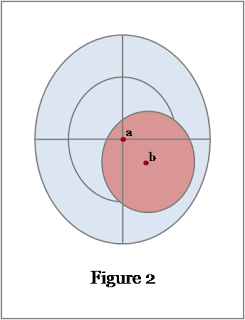## Friday, 1 May 2009

### Error and uncertainty in analytical measurement - Part I

ANALYTICAL TOPICS

When analysing pharmaceuticals the objective of our efforts is to produce a result, an analytical measurement, which gives important information about the sample of interest. Examples are: the determination of impurities in an active pharmaceutical ingredient; and the assay of the amount of the active in a dosage form. In these examples the information obtained may be used to ensure that the product is safe and of high quality. It is vital that the analytical results obtained are reliable since they are used to make decisions which can have an impact on human health.

A reliable result does not mean that the analytical measurement is exactly equal to the actual value of the parameter being measured (the true value). We accept that the result is an estimation of the true value and that the difference is due to error and uncertainty introduced during the measurement process. However, for the result to be deemed reliable, we need to determine the magnitude of this difference between the analytical measurement and the true value. This requires a quantification of the error and/or uncertainty associated with the analytical method.

So what are error and uncertainty, and what is the difference between them?Error is defined by ISO  as “the result of a measurement minus a true value of the measurand”, (where the measurand is the “particular quantity subject to measurement”). In Figure 1 the true value of an analytical measurand is shown by a, the centre of the target. An analytical measurement is shown by b. The difference between the true value and the measured value is given by x. Therefore the error for the measurement is equal to x. Error is a single value for each analytical measurement. In theory a known value for error can be used to correct the result.

Error in analytical measurement is regarded as being made up of two different types: random error and systematic error. Random error is caused by the many uncontrollable variables that are an inevitable part of every chemical or physical measurement. This type of error can usually be reduced by multiple measurements. Systematic errors have a definite cause and are of the same value for a number of replicates analysed in the same way. They are independent of the number of measurements and therefore cannot be reduced by increasing the number of measurements. A further type of error is a spurious error, or blunder, which invalidates a measurement. This can occur when the person performing the analysis makes a mistake or an instrument fails.Uncertainty is defined by ISO  as a “parameter associated with the result of a measurement, that characterises the dispersion of the values that could reasonably be attributed to the measurand.” This parameter may take the form of some multiple of the standard deviation, or confidence limits relating to an analytical measurement. It is usually expressed as a range within which the true value is known to fall. The uncertainty may be determined for a particular analytical method or procedure and thus applied to all results obtained for the method, provided that it is applied in the same way each time.

In Figure 2 the true value of an analytical measurand is again shown by a, the centre of the target and an analytical measurement is again shown by b. The uncertainty of the analytical measurement b is shown by the red shading. The true value is contained within this area, or range of values.Figure 3 illustrates the difference between error and uncertainty. As previously, the true value of an analytical measurand is shown by a at the centre of the target. This time an analytical measurement, c, is shown. This result has been determined using the same analytical method as in Figure 2 and thus has an associated uncertainty shown by the red shading. The difference between a and c is very small and thus the error for this measurement is very small. The analyst knows that the true value lies within the area of red shading but has no way of knowing just how close the measurement is to the true value. In practice it is usually possible to quantify the uncertainty for an analytical procedure, and thus a range within which the true value lies, but quantification of the error for each analytical measurement is not usually possible.

Now that we have defined error and uncertainty, and the distinction between them, we can move on to the sources of error and uncertainty in pharmaceutical analysis and how they may be quantified. This will be discussed in the next issue of Analytical Chemistry Revision Notes, Error and uncertainty in analytical measurement - Part II.

References:
1. International Vocabulary of basic and general terms in Metrology. ISO, Geneva, (1993). (ISBN 92-67-10175-1).
These definitions are reproduced in the publication: S.L.R. Ellison, M. Rösslein, A. Williams, (Eds.), EURACHEM/CITAC Guide, Quantifying Uncertainty in Analytical Measurement, second ed., 2000. http://www.measurementuncertainty.org/mu/guide. This publication has the advantage of being freely available online.

1.Patrícia Gonçalves12 January 2011 at 11:29

Hi, Oona!

Is there a Part II post? I can´t find it...

Thanks,

Patrícia

2.Part II was planned but for some reason I never got round to posting it. Hopefully should be available soon!